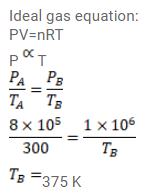# Use R=8.3 J/mol-K wherever required.Question:

Use $\mathrm{R}=8.3 \mathrm{~J} / \mathrm{mol}-\mathrm{K}$ wherever required.

A gas cylinder has walls that can bear a maximum pressure of $1.0^{\times 10^{6}}$ Pa. It contains a gas at $8.0$ $\times 10^{5} \mathrm{~Pa}$ and $300 \mathrm{~K}$. The cylinder is steadily heated. Neglecting any change in the volume, calculate the temperature at which the cylinder will break.

Solution: